# Micro Motion Mass Flow Meter not Working (2700 Transmitters Display)

Mass Flow Measurement Unit = USGPM (US gal/min)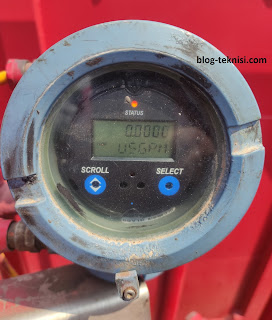Figure 1.1 - Micro Motion 2700 Transmitters

#Configure Process Measurement
1). Start Prolink, and Connect to Physical Device. Read More: Connect to Micro Motion using Service Port and Modbus RTU (8-bit) - Prolink III.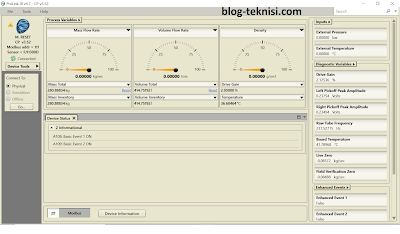Figure 1.2 - Process Variables (F/H300D)

2). Configure Mass Flow Measurement.
The mass flow measurement parameters control how mass flow is measured, specifies the unit of measure that will be used for the mass flow rate.
→Set Mass Flow Measurement Unit to the unit you want to use. In this case, we will use the USGPM (US gal/min), refer to Figure 1.1 above.
→Select Process Variables - Configuration - Flow.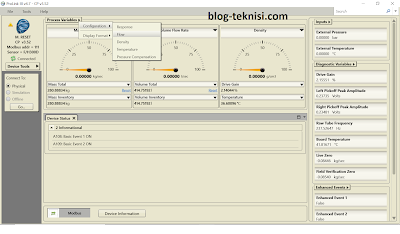Figure 1.3 - Configuration (Flow)
→Select Mass Flow Rate - Mass Flow Rate Unit.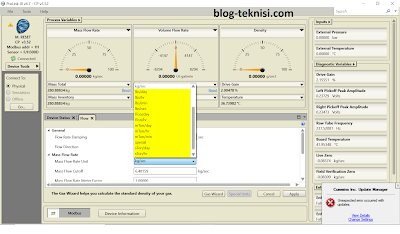Figure 1.4 - Mass Flow Rate Unit
→Select Mass Flow Rate Unit to lbs/min (pounds per minute). Note: a pound of water is equivalent to that of 0.12 gallons (converts 1 pound to gallons).
→Select Apply, and OK to message box displays.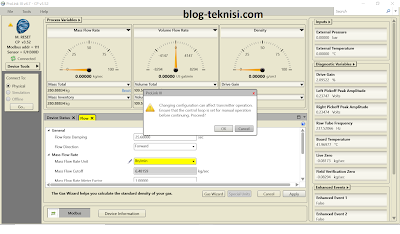Figure 1.5 - Select OK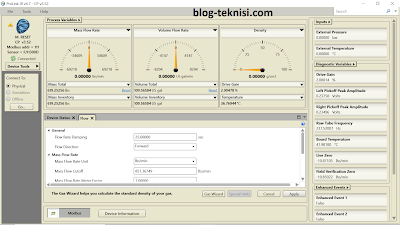Figure 1.6 - Mass Flow Rate (lbs/min)
→Select Volume Flow Rate - Volume Flow Rate Unit.
→Select Volume Flow Rate Unit to US gal/min (USGPM).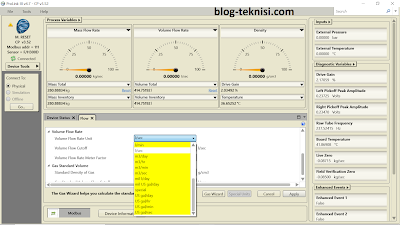Figure 1.7 - Volume Flow Rate Unit
→Select Apply, and OK to message box displays.Figure 1.8 - Select OK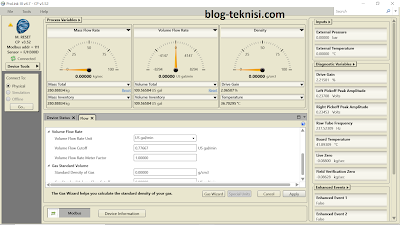Figure 1.9 - Volume Flow Rate Unit (US gal/min)

3). Problem solved: after changed the Mass Flow Rate Unit and Volume Flow Rate Unit.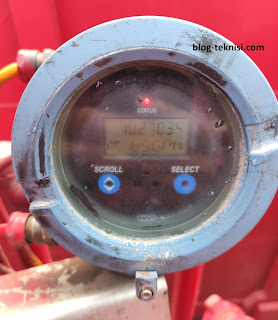Figure 2.1 - Flow 102.70 USGPM